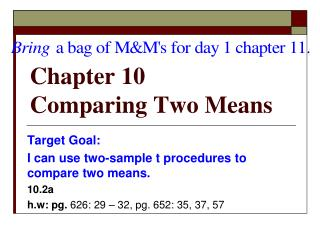DownloadDownload PresentationChapter 10 Comparing Two Means

# Chapter 10 Comparing Two Means

Télécharger la présentation## Chapter 10 Comparing Two Means

- - - - - - - - - - - - - - - - - - - - - - - - - - - E N D - - - - - - - - - - - - - - - - - - - - - - - - - - -
##### Presentation Transcript

1. Chapter 10Comparing Two Means Target Goal: I can use two-sample t procedures to compare two means. 10.2a h.w: pg. 626: 29 – 32, pg. 652: 35, 37, 57

2. Two-Sample Problems • Comparative studies are more convincing than single single-sample investigations, so one-sample inference (for matched pairs) is not as common as comparative (two-sample) inference.

3. In a comparative study:the units or sample sizes can be different. 1) We may want to compare two treatments, or we may want to compare two populations. 2) The samples must be chosen randomlyand independentlyin order to perform statistical inference. • Because match pairs are NOT chosen independently, we will NOT use two-sample inference for a matched pairs design. • Recall: for a matched pairs design, apply the one-sample t procedures to the observed differences.

4. Otherwise, we may use two-sample inference to compare two treatments or two populations.

5. Significance Tests for µ1 – µ2 • The null hypothesis is that there is no difference between the two parameters. • The alternative hypothesis could be that

6. Before you begin, check your assumptions! Conditions for Comparing Two Means Both samples must be a • Random sample or randomized experiment. • must be chosen independently • both populations must be normally distributed. (Check the data for outliers or skewness.)

7. Confidence Intervals for µ1 – µ2.On page 634 Comparing Two Means Two-Sample tInterval for a Difference Between Means

8. Confidence Intervals for µ1 – µ2.On page 634 Comparing Two Means Two-Sample tInterval for a Difference Between Means

9. If these assumptions hold, then the difference in sample means is an unbiased estimator of the difference in population means, so is equal to .

10. The variance of is the sum of the variances of and , which is Note: variances add, standard deviations don’t. Furthermore, if both populationsare normally distributed, then is also normally distributed.

11. Standardizing • Subtract the mean and divide by the standard deviation:

12. If we do not know and , we will substitute with the standard error SE using and for the standard deviation. • This gives the standardized tvalue:

13. Ex: Calcium and blood pressure • Does increasing the amount of calcium in our diet reduce blood pressure? The subjects were 21 healthy black men. A randomly chosen group of 10 received calcium supplement for 12 weeks. A control group of 11 men received a placebo (double blinded experiment). • The response variable is the decrease in systolic (heart contracted) blood pressure. An increase appears as a negative response (drop in blood pressure).

14. Group 1: 7, -4, 18, 17, -3, -5,1,10,11,-2 Group 2: -1, 12, -1,-3, 3, -5, 5, 2, -11, -1, -3 • Calculate summary statistics: (Enter data into lists.) Group Treatment n s 1 Calcium 2 Placebo 10 5.000 8.743 11 -0.273 5.901 Is this outcome good evidence that calcium decreases blood pressure? Perform a significance test.

15. Step 1. State - Identify the population of interest and the parameter you want to draw a conclusion about. State the null and alternative hypothesis in words and symbols. : The mean decrease in blood pressure for those taking calcium is the same as the mean decrease in blood pressure for those taking a placebo. : The mean decrease in blood pressure for those taking calcium is greater than the mean decrease in blood pressure for those taking a placebo.

16. Step 2. Plan –If the conditions are met, we will construct a two-sample t test for Verify the conditions (random, indep, normal). • Random: Because of randomization, we mustassume the calcium and placebo groups as SRS. • Normal: Examine the stem plot in notes. Are there outliers?

17. Step 2. Plan –If the conditions are met, we will construct a two-sample t test for • Normal: Examine the stem plot in notes. Are there outliers? no

18. Are there any departures from normality that would prevent us from using a t-test? Calcium is a little irregular but this is not unusual for a small sample. • Independent: Yes, due to random assignment, the populations can be viewed as independent. Individual observations in each group should also be independent. • Proceed with t procedures.

19. Step 3.Carry out the inference procedure. Compute the test statistic and P-value • To test compute: = 1.604

20. For df, use the smaller of n1 – 1, n2 – 1. There are 9 df, • Find P • tcdf( P = .072

21. Step 4:Interpret your results: • The experiment found evidence that calcium reduces blood pressure but not at the traditional 5% and 1% levels as the p value is .071. • We fail to reject Ho at these levels.

22. We will use graphing calculators to perform two-sample T tests! • 2-SampTTest for a hypothesis test • 2-SampTInt for a confidence interval Confirm the P-value and construct a 90% confidence interval • STAT:TESTS: • Pooled: no • Use data if you have it or Stats if you are given

23. CI = (-.4767, 11.022). • The 90 % C.I. covers 0 (both neg. and pos. changes). • We can not reject Ho against the two sided alternative at the α = 0.10 level of significance. • Read pg. 625 - 637